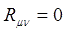## Pages

### 15. Schwarzschild Solution

The Schwarzschild solution is named in honor of Karl Schwarzschild, who found the exact solution in 1916, a little more than a month after the publication of Einstein's theory of general relativity. It was the first exact solution of the Einstein field equations other than the trivial flat space solution. The Schwarzschild solution is one of the simplest and most useful solutions of the Einstein field equations. It describes spacetime in the vicinity of a non-rotating massive spherically-symmetric object.

We have thus written the Field equation in terms of the Ricci tensor and the energy-momentum tensor. In this form, we can immediately make out that in the vicinity of the gravitating matter, (i.e. the empty space) we can reduce the above equation as follows,$(1)$
Now, although equation $(1)$ seems decisively simple, it is not as simple as it looks. We should remember that the symbol $R_{\mu\nu}$ stands for Ricci tensor which is a function of the Christoffel symbol and its derivatives. Moreover, Christoffel symbol is a function of the derivative of the metric tensors too. So, equation $(1)$ is only a short hand notation for a very complex equation indeed.

This equation is also known as the Einstein vacuum field equation and the particular solution found by applying the Schwarzschild assumptions, we solve the equation. We will do that in the next note.